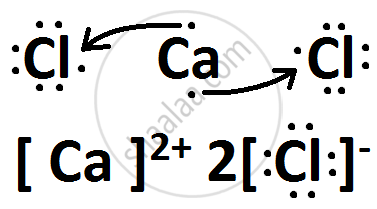# Consider two elements 'X' (Atomic number 17) and 'Y' (Atomic number 20) - Science

Consider two elements 'X' (Atomic number 17) and 'Y' (Atomic number 20)

(i) Write the positions of these elements in the modern periodic table giving justification.

(ii) Write the formula of the compound formed by the combination of 'X' and 'Y'.

(iii) Draw the electron-dot structure of the compound formed and state the nature of the bond formed between the two elements ?

#### Solution

(i) X belongs to Group 17 and 3rd Period.
Y belongs to Group 2 and 4th Period.

(iii) X is Chlorine while Y is Calcium.Concept: Periodic Properties
Is there an error in this question or solution?
2013-2014 (March) Delhi Set 3

Share